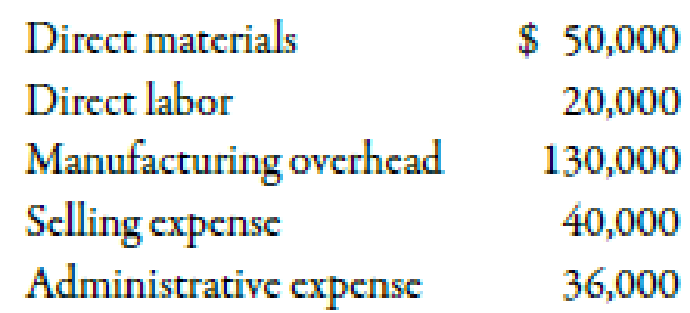# Use the following information for Multiple-Choice Questions 2-13 through 2-18: Last year, Barnard Company incurred the following costs: Barnard produced and sold 10,000 units at a price of $31 each. 2-14 Refer to the information for Barnard Company above. Conversion cost per unit is a.$7.00. b. $20.00. c.$15.00. d. $5.00. e.$27.60.### Managerial Accounting: The Corners...

7th Edition
Maryanne M. Mowen + 2 others
Publisher: Cengage Learning
ISBN: 9781337115773### Managerial Accounting: The Corners...

7th Edition
Maryanne M. Mowen + 2 others
Publisher: Cengage Learning
ISBN: 9781337115773

#### Solutions

Chapter
Section
Chapter 2, Problem 14MCQ
Textbook Problem
188 views

## Use the following information for Multiple-Choice Questions 2-13 through 2-18:Last year, Barnard Company incurred the following costs:Barnard produced and sold 10,000 units at a price of $31 each.2-14 Refer to the information for Barnard Company above. Conversion cost per unit is a.$7.00. b. $20.00. c.$15.00. d. $5.00. e.$27.60.

Expert Solution
To determine

Calculate the amount of conversion cost per unit.

### Explanation of Solution

Conversion Cost Per Unit:

Conversion cost per unit can be defined as the ratio of conversion cost to the number of units produced and sold. Conversion cost can be defined as the summation of direct labor cost and manufacturing overhead.

c.

Use the following formula to calculate the conversion cost per unit:

Conversion cost per unit=Conversion costUnits produced and sold

Substitute $150,000 for conversion cost and 10,000 units for units produced and sold in the above formula. Conversion cost per unit=$150,00010,000units=$15 The conversion cost per unit is$15

### Want to see the full answer?

Check out a sample textbook solution.See solution

### Want to see this answer and more?

Bartleby provides explanations to thousands of textbook problems written by our experts, many with advanced degrees!

See solution